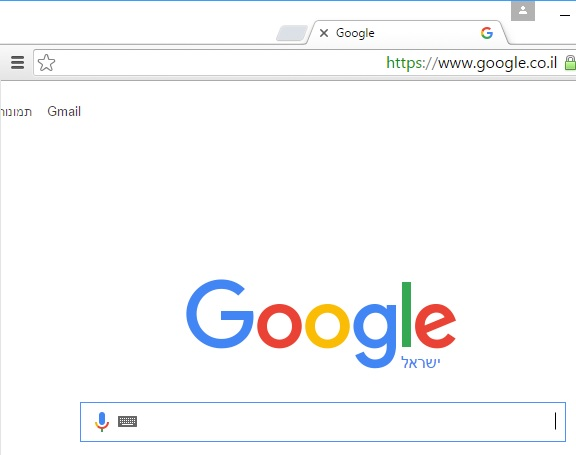# 关于性能：C# 更新图片框中的位图

## C# Update bitmap in picturebox

 12345678910111213141516171819202122232425262728 private void MainScreenThread()     {         ReadData();//reading data from socket.         initial = bufferToJpeg();//first intial full screen image.         pictureBox1.Paint += pictureBox1_Paint;//activating the paint event.         while (true)         {             int pos = ReadData();             x = BlockX();//where to draw :X             y = BlockY();//where to draw :Y             Bitmap block = bufferToJpeg();//constantly reciving blocks.             Draw(block, new Point(x, y));//applying the changes-drawing the block on the big initial image.using native memcpy.             this.Invoke(new Action(() =>             {                 pictureBox1.Refresh();//updaing the picturebox for seeing results.                 // this.Text = ((pos / 1000).ToString() +"KB");             }));         }     }     private void pictureBox1_Paint(object sender, PaintEventArgs e)     {         lock (initial)         {             e.Graphics.DrawImage(initial, pictureBox1.ClientRectangle); //draws at picturebox's bounds         }     }

 1234567891011121314151617181920212223242526272829 private unsafe void Draw(Bitmap bmp2, Point point)     {         lock (initial)         {               BitmapData bmData = initial.LockBits(new Rectangle(0, 0, initial.Width, initial.Height), System.Drawing.Imaging.ImageLockMode.WriteOnly, initial.PixelFormat);             BitmapData bmData2 = bmp2.LockBits(new Rectangle(0, 0, bmp2.Width, bmp2.Height), System.Drawing.Imaging.ImageLockMode.ReadOnly, bmp2.PixelFormat);             IntPtr scan0 = bmData.Scan0;             IntPtr scan02 = bmData2.Scan0;             int stride = bmData.Stride;             int stride2 = bmData2.Stride;             int Width = bmp2.Width;             int Height = bmp2.Height;             int X = point.X;             int Y = point.Y;             scan0 = IntPtr.Add(scan0, stride * Y + X * 3);//setting the pointer to the requested line             for (int y = 0; y < Height; y++)             {                 memcpy(scan0, scan02 ,(UIntPtr)(Width * 3));//copy one line                 scan02 = IntPtr.Add(scan02, stride2);//advance pointers                 scan0 = IntPtr.Add(scan0, stride);//advance pointers//             }             initial.UnlockBits(bmData);             bmp2.UnlockBits(bmData2);         }     }123456789101112131415161718192021222324252627282930313233343536373839404142434445464748 private Rectangle GetViewRect() { return pictureBox1.ClientRectangle; } private void MainScreenThread() {     ReadData();//reading data from socket.     initial = bufferToJpeg();//first intial full screen image.     pictureBox1.Paint += pictureBox1_Paint;//activating the paint event.     // The update action     Action updateAction = imageRect =>     {         var viewRect = GetViewRect();         var scaleX = (float)viewRect.Width / initial.Width;         var scaleY = (float)viewRect.Height / initial.Height;         // Make sure the target rectangle includes the new block         var targetRect = Rectangle.FromLTRB(             (int)Math.Truncate(imageRect.X * scaleX),             (int)Math.Truncate(imageRect.Y * scaleY),             (int)Math.Ceiling(imageRect.Right * scaleX),             (int)Math.Ceiling(imageRect.Bottom * scaleY));         pictureBox1.Invalidate(targetRect);         pictureBox1.Update();     };     while (true)     {         int pos = ReadData();         x = BlockX();//where to draw :X         y = BlockY();//where to draw :Y         Bitmap block = bufferToJpeg();//constantly reciving blocks.         Draw(block, new Point(x, y));//applying the changes-drawing the block on the big initial image.using native memcpy.         // Invoke the update action, passing the updated block rectangle         this.Invoke(updateAction, new Rectangle(x, y, block.Width, block.Height));     } } private void pictureBox1_Paint(object sender, PaintEventArgs e) {     lock (initial)     {         var viewRect = GetViewRect();         var scaleX = (float)initial.Width / viewRect.Width;         var scaleY = (float)initial.Height / viewRect.Height;         var targetRect = e.ClipRectangle;         var imageRect = new RectangleF(targetRect.X * scaleX, targetRect.Y * scaleY, targetRect.Width * scaleX, targetRect.Height * scaleY);         e.Graphics.DrawImage(initial, targetRect, imageRect, GraphicsUnit.Pixel);     } }

 12345678910 ReadData(); initial = bufferToJpeg(); pictureBox1.Image = initial; var graphics = pictureBox1.CreateGraphics(); while (true) {     int pos = ReadData();     Bitmap block = bufferToJpeg();     graphics.DrawImage(block, BlockX(), BlockY()); }